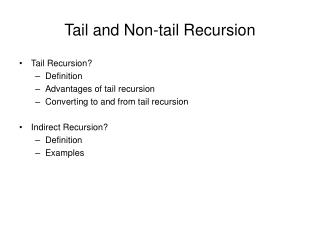DownloadDownload PresentationTail and Non-tail Recursion

# Tail and Non-tail Recursion

Télécharger la présentation## Tail and Non-tail Recursion

- - - - - - - - - - - - - - - - - - - - - - - - - - - E N D - - - - - - - - - - - - - - - - - - - - - - - - - - -
##### Presentation Transcript

1. Tail and Non-tail Recursion • Tail Recursion? • Definition • Advantages of tail recursion • Converting to and from tail recursion • Indirect Recursion? • Definition • Examples

2. What is Tail Recursion? • Recursive methods are either • Tail recursive • Nontail recursive • Tail recursive method has the recursive call as the last statement in the method. • Recursive methods that are not tail recursive are called non-tail recursive

3. Is Factorial Tail Recursive? • Is the factorial method a tail recursive method? int fact(int x){ if (x==0) return 1; else return x*fact(x-1); } • When returning back from a recursive call, there is still one pending operation, multiplication. • Therefore, factorial is a non-tail recursive method.

4. Another Example • Is this method tail recursive? void tail(int i) { if (i>0) { system.out.print(i+"") tail(i-1) } It is tail recursive!

5. Third Example • Is the following program tail recursive? void non prog(int i) { if (i>0) { prog(i-1); System.out.print(i+""); prog(i-1); } } • No, because there is an ealier recursive call, other than the last one, • In tail recursion, the recursive call should be the last statement, and there should be no earlier recursive calls whether direct or indirect.

6. Advantage of Tail Recursive Method • Tail Recursive methods are easy to convert to iterative. • Smart compilers can detect tail recursion and convert it to iterative to optimize code • Used to implement loops in languages that do not support loop structures explicilty (e.g. prolog) void tail(int i){ if (i>0) { system.out.println(i+""); tail(i-1) } } void iterative(int i){ for (;i>0;i--) System.out.println(i+""); }

7. Converting Non-tail to Tail Recursive • A non-tail recursive method can be converted to a tail-recursive method by means of an "auxiliary" parameter used to form the result. • The technique is usually used in conjunction with an "auxiliary" function. This is simply to keep the syntax clean and to hide the fact that auxiliary parameters are needed. int fact_aux(int n, int result) { if (n == 1) return result; return fact_aux(n - 1, n * result) } int fact(n) { return fact_aux(n, 1); }

8. auxiliary parameters! Converting Non-tail to Tail Recursive • A tail-recursive Fibonacci method can be implemented by using two auxiliary parameters for accumulating results. int fib_aux ( int n , int next, int result) { if (n == 0) return result; return fib_aux(n - 1, next + result, next); } To calculate fib(n) , call fib_aux(n,1,0)

9. Converting Tail Recursive to Iterative F(x) { if (P(x)) return G(x); return F(H(x)); } • P(x) is true, the value of F(x) is the value of some other function G(x). Otherwise, the value of F(x) is the value of the function F on some other value, H(x) F(x) { int temp_x = x; while (P(x) is not true) { temp_x = x; x = H(temp_x); } return G(x); }

10. Converting Tail Recursive to Iterative int fact_aux(int n, int result) { if (n == 1) return result; return fact_aux(n - 1, n * result); } F(x) { if (P(x)) return G(x); return F(H(x)); } the function F is fact_aux x is composed of the two parameters, n and result the value of P(n, result) is the value of (n == 1) the value of G(n, result) is result the value of H(n, result) is (n -1, n * result) int fact_iter(int n, int result) { int temp_n; int temp_result; while (n != 1) { temp_n = n; temp_result = result; n = temp_n - 1; result = temp_n * temp_result; } return result; } F(x) { int temp_x = x; while (P(x) is not true) { temp_x = x; x = H(temp_x); } return G(x); }

11. Indirect Recursion • If X makes a recursive call to X itself, it is called direct recursion. • Indirect recursive methods call themselves indirectly through calling other methods. • In general, indirect recursion is a circular sequence of two or more recursive calls: X1 --> X2 --> ... --> X1.

12. Indirect Recursion Example • Three method for decoding information are: • receive: stores information in buffer and calls decode. • decode: converts information to new form and calls store • store : stored information in a file, call receive. • We have a chain of calls: recieve() -> decode() -> store() ->recieve()-> decode()....

13. Example: Information Decoding recieve (buffer) while buffer is not filled up if information is still incoming get a character and store it in buffer; else exit() decode (buffer); decode(buffer) decode information in buffer; store (buffer); store(buffer) transfer information from buffer to file; recieve (buffer);

14. Indirect Recursion:Another Example • Determine whether an integer is even or odd: • How do we know if a number is even? • we know 0 is even. • we know that if n is even, then n-1 must be odd. static boolean is_even(int n) { if (n==0) return true; else return(is_odd(n-1)); } • How do we know if a number is odd? It's not even! tatic boolean is_odd(int n) { return (!is_even(n)); }